# Variational Autoencoder with Tensorflow 2.8 – VII – KL loss via model.add_loss()

I continue my series on options regarding the treatment of the Kullback-Leibler divergence as a loss [KL loss] in Variational Autoencoder [VAE] setups.

Our objective is to find solutions which avoid potential problems with the eager execution mode of present Tensorflow 2 implementations. Popular recipes of some teaching books on ML may lead to non-working codes in present TF2 environments. We have already looked at two working alternatives.

In the last post we transferred the „mu“ and „log_var“ tensors from the Encoder to the Decoder and fed some Keras standard loss functions with these tensors. These functions could in turn be inserted into the model.compile() statement. The approach was a bit complex because it involved multi-input-output model definitions for the Encoder and Decoder.

The present article will discuss a third and lighter approach – namely using the Keras add_loss() mechanism on the level of a Keras model, i.e. model.add_loss().

The advantage of this function is that its parameter interface is not reduced to the form of standardized Keras cost function interfaces which I used in my last post. This gives us flexibility. A solution based on model.add_loss() is also easy to understand and realize on the programming level. It is, however, an approach which may under certain conditions reduce performance by roughly a factor between 1.3 and 1.5 – which is significant. I admit that I have not yet understood what the reasons are. But the concrete solution version I present below works well.

# The strategy

The way how to use Keras‘ add_loss() functionality is described in the Keras documentation. I quote from this part of TF2’s documentation about the use of add_loss():

This method can also be called directly on a Functional Model during construction. In this case, any loss Tensors passed to this Model must be symbolic and be able to be traced back to the model’s Inputs. These losses become part of the model’s topology and are tracked in get_config.

The documentation also contains a simple example. The strategy is to first define a full VAE model with standard mu and log_var layers in the Encoder part – and afterwards add the KL-loss to this model. This is depicted in the following graphics: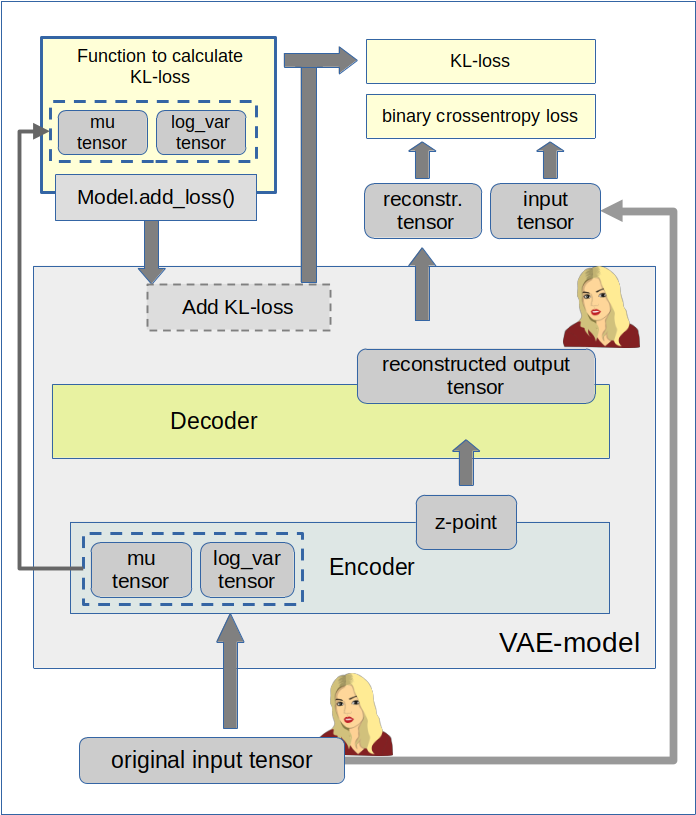We implement this strategy below via the Python class for a VAE setup which we have used already in the last 4 posts of this series. We control the Keras model setup and the layer construction by the parameter „solution_type“, which I have introduced in my last post.

# Cosmetic changes to the Encoder/Decoder parts and the model creation

The class method _build_enc(self, …) can remain as it was defined in the last post. We just have to change the condition for the layer setup as follows:

Change to _build_enc(self, …)

```... # see other posts
...
# The Encoder Model
# ~~~~~~~~~~~~~~~~~~~
# With extra KL layer or with vae.add_loss()
if solution_type == 0 or solution_type == 2:
self.encoder = Model(self._encoder_input, self._encoder_output)

# Transfer solution => Multiple outputs
if solution_type == 1:
self.encoder = Model(inputs=self._encoder_input, outputs=[self._encoder_output, self.mu, self.log_var], name="encoder")
```

Something similar holds for the Decoder part _build_decoder(…):

Change to _build_dec(self, …)

```... # see other posts
...
# The Decoder model
# solution_type == 0/2: Just the decoded input
if self.solution_type == 0 or self.solution_type == 2:
self.decoder = Model(self._decoder_inp_z, self._decoder_output)

# solution_type == 1: The decoded tensor plus the transferred tensors mu and log_var a for the variational distribution
if self.solution_type == 1:
self.decoder = Model([self._decoder_inp_z, self._dec_inp_mu, self._dec_inp_var_log],
[self._decoder_output, self._dec_mu, self._dec_var_log], name="decoder")
```

A similar change is done regarding the model definition in the method _build_VAE(self):

Change to _build_VAE(self)

```        solution_type = self.solution_type

if solution_type == 0 or solution_type == 2:
model_input  = self._encoder_input
model_output = self.decoder(self._encoder_output)
self.model = Model(model_input, model_output, name="vae")

... # see other posts
...
```

# Changes to the method compile_myVAE(self, learning_rate)

More interesting is a function which we add inside the method compile_myVAE(self, learning_rate, …).

Changes to compile_myVAE(self, learning_rate):

```    # Function to compile the full VAE
# ~~~~~~~~~~~~~~~~~~~~~~~~~~~~~~~~~~~~~~~~~~~~~
def compile_myVAE(self, learning_rate):

# Optimizer
# ~~~~~~~~~
# save the learning rate for possible intermediate output to files
self.learning_rate = learning_rate

# Parameter "fact" will be used by the cost functions defined below to scale the KL loss relative to the BCE loss
fact = self.fact

# Function for solution_type == 1
# ~~~~~~~~~~~~~~~~~~~~~~~~~~~~~~~~~
@tf.function
def mu_loss(y_true, y_pred):
loss_mux = fact * tf.reduce_mean(tf.square(y_pred))
return loss_mux

@tf.function
def logvar_loss(y_true, y_pred):
loss_varx = -fact * tf.reduce_mean(1 + y_pred - tf.exp(y_pred))
return loss_varx

# Function for solution_type == 2
# ~~~~~~~~~~~~~~~~~~~~~~~~~~~~~~~~
# We follow an approach described at
# https://www.tensorflow.org/api_docs/python/tf/keras/layers/Layer
# NOTE: We can NOT use @tf.function here
def get_kl_loss(mu, log_var):
kl_loss = -fact * tf.reduce_mean(1 + log_var - tf.square(mu) - tf.exp(log_var))
return kl_loss

# Required operations for solution_type==2 => model.add_loss()
# ~~~~~~~~~~~~~~~~~~~~~~~~~~~~~~~~~~~~~~~~~
res_kl = get_kl_loss(mu=self.mu, log_var=self.log_var)
if self.solution_type == 2:

# Model compilation
# ~~~~~~~~~~~~~~~~~~~~
if self.solution_type == 0 or self.solution_type == 2:
self.model.compile(optimizer=optimizer, loss="binary_crossentropy",
metrics=[tf.keras.metrics.BinaryCrossentropy(name='bce')])

if self.solution_type == 1:
self.model.compile(optimizer=optimizer
, loss={'vae_out_main':'binary_crossentropy', 'vae_out_mu':mu_loss, 'vae_out_var':logvar_loss}
#, metrics={'vae_out_main':tf.keras.metrics.BinaryCrossentropy(name='bce'), 'vae_out_mu':mu_loss, 'vae_out_var': logvar_loss }
)
```

I have supplemented function get_kl_loss(mu, log_var). We explicitly provide the tensors „self.mu“ and „self.log_var“ via the function’s interface and thus follow one of our basic rules for the Keras add_loss()-functionality (see post IV).
Note that this is a MUST to get a working solution for eager execution mode!

Interestingly, the flexibility of model.add_loss() has a price, too. We can NOT use a @tf.function indicator here – in contrast to the standard cost functions which we used in the last post.

Note also that I have added some metrics to get detailed information about the size of the crossentropy-loss and the KL-loss during training!

# Cosmetic change to the method for training

Eventually we must include solution_type==2 in method train_myVAE(self, x_train, batch_size, …)

Changes to train_myVAE(self, x_train, batch_size,…)

```... # see other posts
...
if self.solution_type == 0 or self.solution_type == 2:
self.model.fit(
x_train
, x_train
, batch_size = batch_size
, shuffle = True
, epochs = epochs
, initial_epoch = initial_epoch
)

if self.solution_type == 1:
self.model.fit(
x_train
#               Working
#                , [x_train, t_mu, t_logvar] # we provide some dummy tensors here
# by dict:
, {'vae_out_main': x_train, 'vae_out_mu': t_mu, 'vae_out_var':t_logvar}
, batch_size = batch_size
, shuffle = True
, epochs = epochs
, initial_epoch = initial_epoch
#, verbose=1
, callbacks=[MyPrinterCallback()]
)

```

# Some results

We can use a slightly adapted version of the Jupyter notebook cells discussed in post V

Cell 6:

```from my_AE_code.models.MyVAE_2 import MyVariationalAutoencoder

z_dim         = 12
solution_type = 2
fact          = 6.5e-4

vae = MyVariationalAutoencoder(
input_dim = (28,28,1)
, encoder_conv_filters = [32,64,128]
, encoder_conv_kernel_size = [3,3,3]
, encoder_conv_strides = [1,2,2]
, decoder_conv_t_filters = [64,32,1]
, decoder_conv_t_kernel_size = [3,3,3]
, decoder_conv_t_strides = [2,2,1]
, z_dim = z_dim
, solution_type = solution_type
, act   = 0
, fact  = fact
)

```

Cell 11:

```BATCH_SIZE = 256
EPOCHS = 37
PRINT_EVERY_N_BATCHES = 100
INITIAL_EPOCH = 0

if solution_type == 2:
vae.train_myVAE(
x_train[0:60000]
, batch_size = BATCH_SIZE
, epochs = EPOCHS
, initial_epoch = INITIAL_EPOCH
)

```

Note that I have changed the BATCH_SIZE to 256 this time; the performance got a bit better then on my old Nvidia 960 GTX:

```Epoch 3/37
235/235 [==============================] - 10s 44ms/step - loss: 0.1135 - bce: 0.1091 - kl: 0.0044
Epoch 4/37
235/235 [==============================] - 10s 44ms/step - loss: 0.1114 - bce: 0.1070 - kl: 0.0044
Epoch 5/37
235/235 [==============================] - 10s 44ms/step - loss: 0.1098 - bce: 0.1055 - kl: 0.0044
Epoch 6/37
235/235 [==============================] - 10s 43ms/step - loss: 0.1085 - bce: 0.1041 - kl: 0.0044
```

This is comparable to data we got for our previous solution approaches. But see an additional section on performance below.

# Some results

As in the last posts I show some results for the MNIST data without many comments. The first plot proves the reconstruction abilities of the VAE for a dimension z-dim=12 of the latent space.

MNIST with z-dim=12 and fact=6.5e-4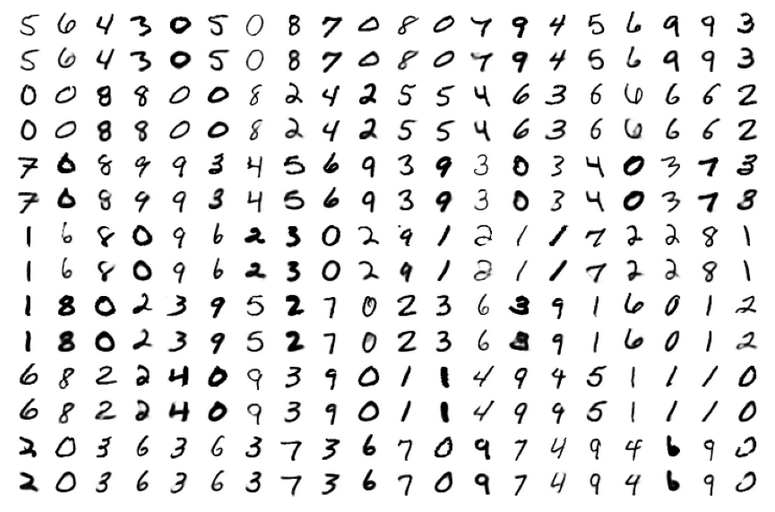For z_dim=2 we get a reasonable data point distribution in the latent space due to the KL loss, but the reconstruction ability suffers, of course:

MNIST with z-dim=2 and fact=6.5e-4 – train data distribution in the z-space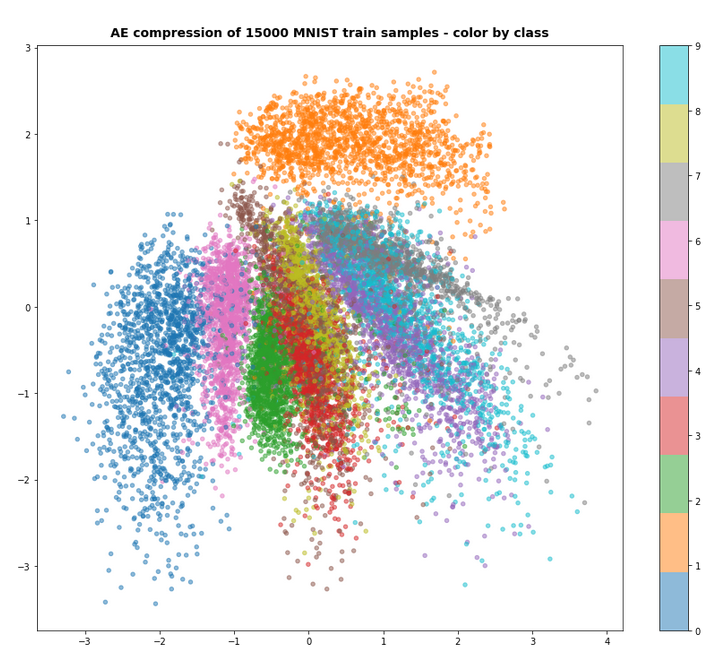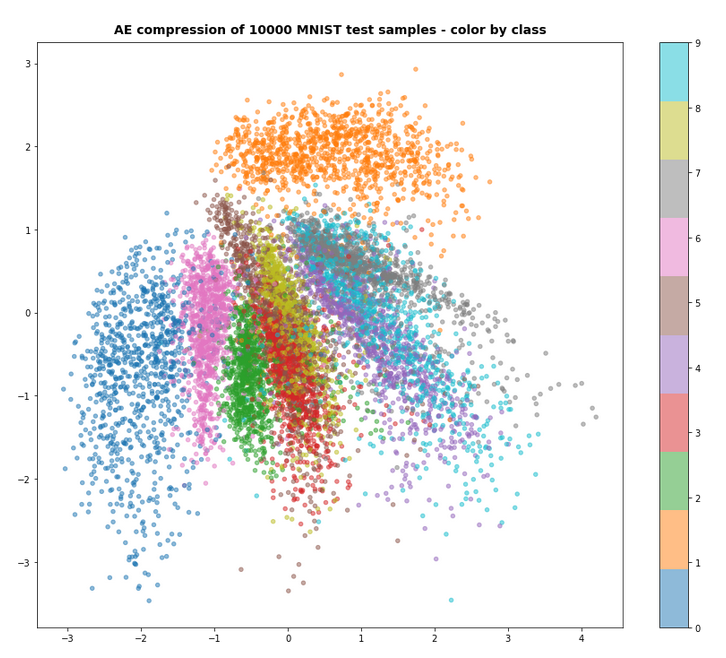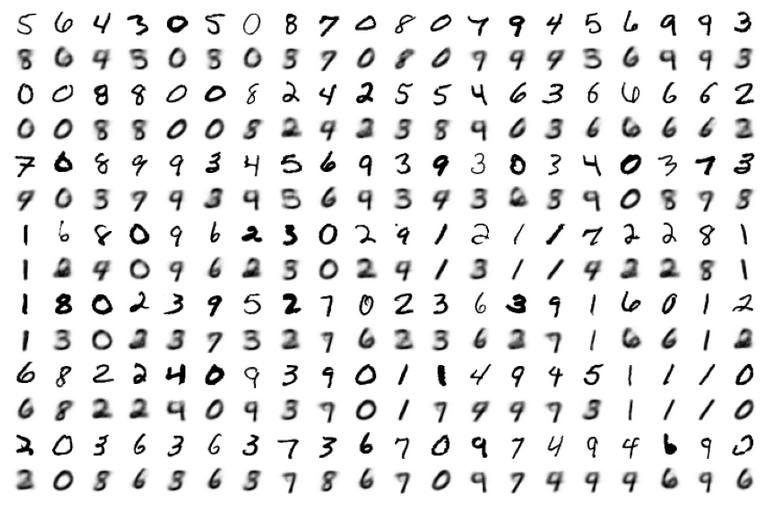For a dimension of z_dim=2 of the latent space and MNIST data we get the following reconstruction chart for data points in a region around the latent space’s origin

# A strange performance problem when no class is used

I also tested a version of the approach with model.add_loss() without encapsulating everything in a class. But with the same definition of the Encoder, the Decoder, the model, etc. But all variables as e.g. mu, log_var were directly kept as data of and in the Jupyter notebook. Then a call

```n_epochs      = 3
batch_size    = 128
initial_epoch = 0
vae.fit( x_train[0:60000],
x_train[0:60000],   # entscheidend !
batch_size=batch_size,
shuffle=True,
epochs = n_epochs,
initial_epoch = initial_epoch
)
```

reduced the performance by a factor of 1.5. I have experimented quite a while. But I have no clue at the moment why this happens and how the effect can be avoided. I assume some strange data handling or data transfer between the Jupyter notebook and the graphics card. I can provide details if some developer is interested.

But as one should encapsulate functionality in classes anyway I have not put efforts in a detail analysis.

# Conclusion

In this article we have studied an approach to handle the Kullback-Leibler loss via the model.add_loss() functionality of Keras. We supplemented our growing class for a VAE with respective methods. All in all the approach is almost more convenient as the solution based on a special layer and layer.add_loss(); see post V.

However, there seems to exist some strange performance problem when you avoid a reasonable encapsulation in a class and do the modell setup directly in Jupyter cells and for Jupyter variables.

In the next post
Variational Autoencoder with Tensorflow 2.8 – VIII – TF 2 GradientTape(), KL loss and metrics
I shall have a look at the solution approach recommended by F. Chollet.

We must provide tensors explicitly to model.add_loss()
https://towardsdatascience.com/shared-models-and-custom-losses-in-tensorflow-2-keras-6776ecb3b3a9

Ceterum censeo: The worst fascist, war criminal and killer living today, who must be isolated, be denazified and imprisoned, is the Putler. Long live a free and democratic Ukraine!

# Variational Autoencoder with Tensorflow 2.8 – VI – KL loss via tensor transfer and multiple output

I continue with my series on options of how to handle the KL loss in Variational Autoencoders [VAEs] in a Tensorflow 2 environment with eager execution:

In the last post we delegated the KL loss calculation to a special customized layer of the Encoder. The layer directly followed two Dense layers which produced the tensors for

• the mean values mu
• and the logarithms of the variances log_var

of statistical standard distributions for z-points in the latent space. (Keep in mind that we have one mu and log_var for each sample. The KL loss function has a compactification impact on the z-point distribution as a whole and a normalization effect regarding the distribution around each z-point.)

The layer centered approach for the KL loss proved to be both elegant and fast in combination with Tensorflow 2. And it fits very well to the way we build ANNs with Keras.

In the present post I focus on a different and more complicated strategy: We shall couple the Encoder and the Decoder by multi-output and multi-input interfaces to transfer mu and log_var tensors to the output side of our VAE model. And then we will calculate the KL loss by using a Keras standard mechanism for costs related to multiple output channels:

We can define a standardized customizable cost function per output channel (= per individual output tensor). Such a Keras cost function accepts two standard input variables: a predicted output tensor for the output channel and a related tensor with true values.

Such costs will automatically be added up to get a total loss and they will be subject to automatic error back propagation under eager execution conditions. However, to use this mechanism requires to transport KL related tensors to the Decoder’s output side and to split the KL loss into components.

The approach is a bit of an overkill to handle the KL loss. But it will also sheds a light on

• multi-in- and multi-output models
• multi-loss models
• and a transfer of tensors between to co-working neural nets.

Therefore the approach is interesting beyond VAE purposes.

Below I will first explain some more details of the present strategy. Afterward we need to find out how to handle standard customized Keras cost-functions for the KL loss contributions and the main loss. Furthermore we have to deal with reasonable output for the different loss terms during the training epochs. A performance comparison will show that the solution – though complicated – is a fast one.

# The strategy in more details: A transfer variational KL tensors from the Encoder to the Decoder

First a general reminder: During training of a Keras model we have to guarantee a correct calculation of partial derivatives of losses with respect to trainable parameters (weights) according to the chain rule. The losses and related tensors themselves depend on matrix operations involving the layers‘ weights and activation functions. So the chain rule has to be applied along all paths through the network. With eager execution all required operations and tensors must already be totally clear during a forward pass to the layers. We saw this already with the solution approach which we discussed in

Variational Autoencoder with Tensorflow 2.8 – IV – simple rules to avoid problems with eager execution

This means that relevant tensors must explicitly be available whenever derivatives shall be handled or pre-defined. This in turn means: When we want to calculate cost contributions after the definition of the full VAE model then we must transfer all required tensors down the line. Wth the functional Keras API we could use them by a direct Python reference to a layer. The alternative is to use them as explicit output of our VAE-model.

The strategy of this post is basically guided by a general Keras rule:

A personally customized cost function which can effortlessly be used in the compile()-statement for a Keras model in an eager execution environment should have a standard interface given by

cost_function( y_true, y_pred )

With exactly these two tensors as parameters – and nothing else!
See https://keras.io/api/losses/#creating-custom-losses. Such a function can be used for each of the multiple outputs of a Keras model.

One reason for this strict rule is that with eager execution the dependence of any function on input variables (tensors) must explicitly be defined via the function’s interface. For a standardized interface of a customizable model’s cost function the necessary steps can be generalized. The advantage of invoking cost functions with standardized interfaces for multiple output channels is, of course, the ease of use.

In the case of an Autoencoder the dominant predicted output is the (reconstructed) output tensor calculated from a z-point by the Decoder. By a comparison of this output tensor (e.g. a reconstructed image) with the original input tensor of the Encoder (e.g. an original image) a value for the binary crossentropy loss can be calculated. We extend this idea about output tensors of the VAE model now to the KL related tensors:

When you look at the KL loss definition in the previous posts with respect to mu and log_var tensors of the Encoder

```kl_loss = -0.5e-4 * tf.reduce_mean(1 + log_var - tf.square(mu) - tf.exp(log_var))
```

you see that we can split it in log_var- and mu-dependent terms. If we could transfer the mu and log_var tensors from the Encoder part to the Decoder part of a VAE we could use these tensors as explicit output of the VAE-model and thus as input for the simple standardized Keras loss functions. Without having to take any further care of eager execution requirements …

So: Why not use

• a multiple-output model for the Encoder, which then provides z-points plus mu and log_var tensors,
• a multiple-input, multiple-output model for the Decoder, which then accepts the multiple output tensors of the Encoder as input and provides a reconstruction tensor plus the mu and log_var tensors as multiple outputs
• and simple customizable Keras cost-functions in the compile() statement for the VAE-model with each function handling one of the VAE’s (= Decoder’s) multiple outputs afterward?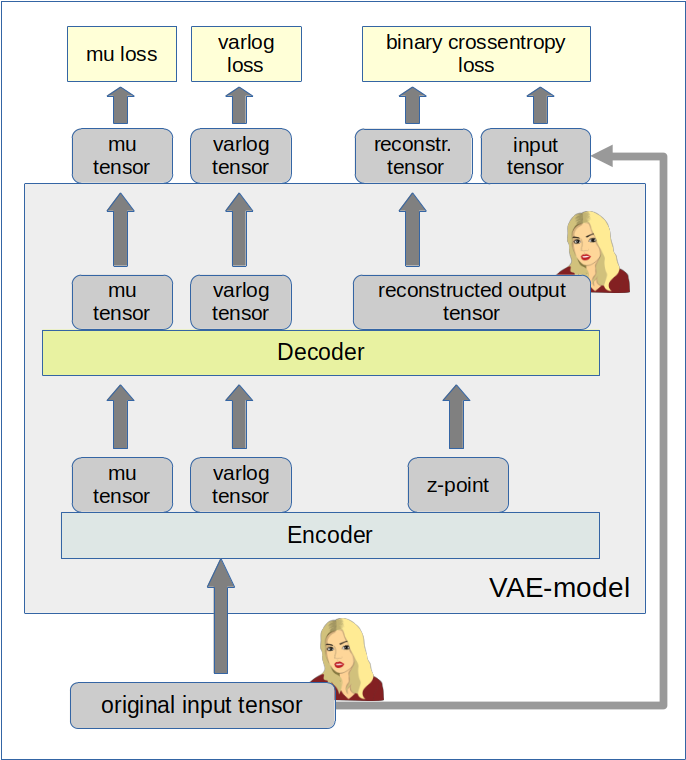# Changes to the class MyVariationalAutoencoder

In the last post I have already described a class which handles all model-setup operations. We are keeping the general structure of the class – but we allow now for options in various methods to realize a different solution based on our present strategy. We shall use the input variable „solution_type“ to the __init__() function for controlling the differences. The __init__() function itself can remain as it was defined in the last post.

# Changes to the Encoder

We change the method to build the encoder of the class „MyVariationalAutoencoder“ in the following way:

```    # Method to build the Encoder
# ~~~~~~~~~~~~~~~~~~~~~~~~~~~
def _build_enc(self, solution_type = -1, fact=-1.0):

# Checking whether "fact" and "solution_type" for the KL loss shall be overwritten
if fact < 0:
fact = self.fact
if solution_type < 0:
solution_type = self.solution_type
else:
self.solution_type = solution_type

# Preparation: We later need a function to calculate the z-points in the latent space
# The following function will be used by an eventual Lambda layer of the Encoder
def z_point_sampling(args):
'''
A point in the latent space is calculated statistically
around an optimized mu for each sample
'''
mu, log_var = args # Note: These are 1D tensors !
epsilon = B.random_normal(shape=B.shape(mu), mean=0., stddev=1.)
return mu + B.exp(log_var / 2) * epsilon

# Input "layer"
self._encoder_input = Input(shape=self.input_dim, name='encoder_input')

# Initialization of a running variable x for individual layers
x = self._encoder_input

# Build the CNN-part with Conv2D layers
# Note that stride>=2 reduces spatial resolution without the help of pooling layers
for i in range(self.n_layers_encoder):
conv_layer = Conv2D(
filters = self.encoder_conv_filters[i]
, kernel_size = self.encoder_conv_kernel_size[i]
, strides = self.encoder_conv_strides[i]
, padding = 'same'  # Important ! Controls the shape of the layer tensors.
, name = 'encoder_conv_' + str(i)
)
x = conv_layer(x)

# The "normalization" should be done ahead of the "activation"
if self.use_batch_norm:
x = BatchNormalization()(x)

# Selection of activation function (out of 3)
if self.act == 0:
x = LeakyReLU()(x)
elif self.act == 1:
x = ReLU()(x)
elif self.act == 2:
# RMO: Just use the Activation layer to use SELU with predefined (!) parameters
x = Activation('selu')(x)

# Fulfill some SELU requirements
if self.use_dropout:
if self.act == 2:
x = AlphaDropout(rate = 0.25)(x)
else:
x = Dropout(rate = 0.25)(x)

# Last multi-dim tensor shape - is later needed by the decoder
self._shape_before_flattening = B.int_shape(x)[1:]

# Flattened layer before calculating VAE-output (z-points) via 2 special layers
x = Flatten()(x)

# "Variational" part - create 2 Dense layers for a statistical distribution of z-points
self.mu      = Dense(self.z_dim, name='mu')(x)
self.log_var = Dense(self.z_dim, name='log_var')(x)

if solution_type == 0:
# Customized layer for the calculation of the KL loss based on mu, var_log data
# We use a customized layer according to a class definition
self.mu, self.log_var = My_KL_Layer()([self.mu, self.log_var], fact=fact)

# Layer to provide a z_point in the Latent Space for each sample of the batch
self._encoder_output = Lambda(z_point_sampling, name='encoder_output')([self.mu, self.log_var])

# The Encoder Model
# ~~~~~~~~~~~~~~~~~~~
# With KL -layer
if solution_type == 0:
self.encoder = Model(self._encoder_input, self._encoder_output)

# With transfer solution => Multiple outputs
if solution_type == 1:
self.encoder = Model(inputs=self._encoder_input, outputs=[self._encoder_output, self.mu, self.log_var], name="encoder")
# Other option
#self.enc_inputs = {'mod_ip': self._encoder_input}
#self.encoder = Model(inputs=self.enc_inputs, outputs=[self._encoder_output, self.mu, self.log_var], name="encoder")

```

For our present approach those parts are relevant which depend on the condition „solution_type == 1“.

Hint: Note that we could have used a dictionary to describe the input to the Encoder. In more complex models this may be reasonable to achieve formal consistency with the multiple outputs of the VAE-model which will often be described by a dictionary. In addition the losses and metrics of the VAE-model will also be handled by dictionaries. By the way: The outputs as well the respective cost and metric assignments of a Keras model must all be controlled by the same class of a Python enumerator.

The Encoder’s multi-output is described by a Python list of 3 tensors: The encoded z-point vectors (length: z_dim!), the mu- and the log_var 1D-tensors (length: z_dim!). (Note that the full shape of all tensors also depends on the batch-size during training where these tensors are of rank 2.) We can safely use a list here as we do not couple this output directly with VAE loss functions or metrics controlled by dictionaries. We use dictionaries only in the output definitions of the VAE model itself.

# Changes to the Decoder

Now we must realize the transfer of the mu and log_var tensors to the Decoder. We have to change the Decoder into a multi-input model:

```    # Method to build the Decoder
# ~~~~~~~~~~~~~~~~~~~~~~~~~~~
def _build_dec(self):

# 1st Input layer - aligned to the shape of z-points in the latent space = output of the Encoder
self._decoder_inp_z = Input(shape=(self.z_dim,), name='decoder_input')

# Additional Input layers for the KL tensors (mu, log_var) from the Encoder
if self.solution_type == 1:
self._dec_inp_mu       = Input(shape=(self.z_dim), name='mu_input')
self._dec_inp_var_log  = Input(shape=(self.z_dim), name='logvar_input')

# We give the layers later used as output a name
# Each of the Activation layers below just corresponds to an identity passed through
self._dec_mu            = Activation('linear',name='dc_mu')(self._dec_inp_mu)
self._dec_var_log       = Activation('linear', name='dc_var')(self._dec_inp_var_log)

# Nxt we use the tensor shape info from the Encoder
x = Dense(np.prod(self._shape_before_flattening))(self._decoder_inp_z)
x = Reshape(self._shape_before_flattening)(x)

# The inverse CNN
for i in range(self.n_layers_decoder):
conv_t_layer = Conv2DTranspose(
filters = self.decoder_conv_t_filters[i]
, kernel_size = self.decoder_conv_t_kernel_size[i]
, strides = self.decoder_conv_t_strides[i]
, padding = 'same' # Important ! Controls the shape of tensors during reconstruction
# we want an image with the same resolution as the original input
, name = 'decoder_conv_t_' + str(i)
)
x = conv_t_layer(x)

# Normalization and Activation
if i < self.n_layers_decoder - 1:
# Also in the decoder: normalization before activation
if self.use_batch_norm:
x = BatchNormalization()(x)

# Choice of activation function
if self.act == 0:
x = LeakyReLU()(x)
elif self.act == 1:
x = ReLU()(x)
elif self.act == 2:
#x = self.selu_scale * ELU(alpha=self.selu_alpha)(x)
x = Activation('selu')(x)

# Adaptions to SELU requirements
if self.use_dropout:
if self.act == 2:
x = AlphaDropout(rate = 0.25)(x)
else:
x = Dropout(rate = 0.25)(x)

# Last layer => Sigmoid output
# => This requires scaled input => Division of pixel values by 255
else:
x = Activation('sigmoid', name='dc_reco')(x)

# Output tensor => a scaled image
self._decoder_output = x

# The Decoder model

# solution_type == 0: Just the decoded input
if self.solution_type == 0:
self.decoder = Model(self._decoder_inp_z, self._decoder_output)

# solution_type == 1: The decoded tensor plus
#                     plus the transferred tensors mu and log_var a for the variational distributions
if self.solution_type == 1:
self.decoder = Model([self._decoder_inp_z, self._dec_inp_mu, self._dec_inp_var_log],
[self._decoder_output, self._dec_mu, self._dec_var_log], name="decoder")
```

You see that the Decoder has evolved into a „multi-input, multi-output model“ for „solution_type==1“.

# Construction of the VAE model

Next we define the full VAE model. We want to organize its multiple outputs and align them with distinct loss functions and maybe also some metrics information. I find it clearer to do this via dictionaries, which refer to layer names in a concise way.

```    # Function to build the full VAE
# ~~~~~~~~~~~~~~~~~~~~~~~~~~~~~~~~~~~~~~~~~~~~~
def _build_VAE(self):

solution_type = self.solution_type

if solution_type == 0:
model_input  = self._encoder_input
model_output = self.decoder(self._encoder_output)
self.model = Model(model_input, model_output, name="vae")

if solution_type == 1:
enc_out      = self.encoder(self._encoder_input)
dc_reco, dc_mu, dc_var = self.decoder(enc_out)

# We organize the output and later association of cost functions and metrics via a dictionary
mod_outputs = {'vae_out_main': dc_reco, 'vae_out_mu': dc_mu, 'vae_out_var': dc_var}
self.model = Model(inputs=self._encoder_input, outputs=mod_outputs, name="vae")

# Another option if we had defined a dictionary for the encoder input
#self.model = Model(inputs=self.enc_inputs, outputs=mod_outputs, name="vae")

```

# Compilation and Costs

The next logical step is to define our cost contributions. I am going to do this as with the help of two sub-functions of a method leading to the compilation of the VAE-model.

```    # Function to compile the full VAE
# ~~~~~~~~~~~~~~~~~~~~~~~~~~~~~~~~~~~~~~~~~~~~~
def compile_myVAE(self, learning_rate):
# Optimizer
# save the learning rate for possible intermediate output to files
self.learning_rate = learning_rate

# Parameter "fact" will be used by the cost functions defined below to scale the KL loss relative to the BCE loss
fact = self.fact

#mu-dependent cost contributions to the KL loss
@tf.function
def mu_loss(y_true, y_pred):
loss_mux = fact * tf.reduce_mean(tf.square(y_pred))
return loss_mux

#log_var dependent cost contributions to the KL loss
@tf.function
def logvar_loss(y_true, y_pred):
loss_varx = -fact * tf.reduce_mean(1 + y_pred - tf.exp(y_pred))
return loss_varx

# Model compilation
# ~~~~~~~~~~~~~~~~~~~~
if self.solution_type == 0:
self.model.compile(optimizer=optimizer, loss="binary_crossentropy",
metrics=[tf.keras.metrics.BinaryCrossentropy(name='bce')])

if self.solution_type == 1:
self.model.compile(optimizer=optimizer
, loss={'vae_out_main':'binary_crossentropy', 'vae_out_mu':mu_loss, 'vae_out_var':logvar_loss}
#, metrics={'vae_out_main':tf.keras.metrics.BinaryCrossentropy(name='bce'), 'vae_out_mu':mu_loss, 'vae_out_var': logvar_loss }
)
```

The first interesting thing is that the statements inside the two cost functions ignore „y_true“ completely. Unfortunately, a small test shows that we nevertheless must provide some reasonable dummy tensors here. „None“ is NOT working in this case.

The dictionary organizes the different costs and their relation to the three output channels of our VAE-model. I have included the metrics as a comment for the moment. It would only produce double output and consume a bit of performance.

# A method for training and fit()

To enable training we use the following function:

```    def train_myVAE(self, x_train, batch_size, epochs, initial_epoch = 0, t_mu=None, t_logvar=None ):

if self.solution_type == 0:
self.model.fit(
x_train
, x_train
, batch_size = batch_size
, shuffle = True
, epochs = epochs
, initial_epoch = initial_epoch
)
if self.solution_type == 1:
self.model.fit(
x_train
# , [x_train, t_mu, t_logvar] # we provide some dummy tensors here
, {'vae_out_main': x_train, 'vae_out_mu': t_mu, 'vae_out_var':t_logvar}
, batch_size = batch_size
, shuffle = True
, epochs = epochs
, initial_epoch = initial_epoch
)
```

You may wonder what the „t_mu“ and „t_log_var“ stand for. These are the dummy tensors which have to provide to the cost functions. The fit() function gets „x_train“ as the model’s input. The tensors „y_pred“, for which we optimize, are handed over to the three loss functions by

```{ 'vae_out_main': x_train, 'vae_out_mu': t_mu, 'vae_out_var':t_logvar}
```

Again, I have organized the correct association to each output and loss contribution via a dictionary.

# Testing

We can use the same Jupyter notebook with almost the same cells as in my last post V. An adaption is only required for the cells starting the training.

I build a „vae“ object (which can later be used for the MNIST dataset) by

Cell 6

```from my_AE_code.models.MyVAE_2 import MyVariationalAutoencoder

z_dim = 2
vae = MyVariationalAutoencoder(
input_dim = (28,28,1)
, encoder_conv_filters = [32,64,128]
, encoder_conv_kernel_size = [3,3,3]
, encoder_conv_strides = [1,2,2]
, decoder_conv_t_filters = [64,32,1]
, decoder_conv_t_kernel_size = [3,3,3]
, decoder_conv_t_strides = [2,2,1]
, z_dim = z_dim
, solution_type = 1  # now we must provide the solution type - here the solution with KL tensor Transfer
, act   = 0
, fact  = 1.e-3
)
```

Afterwards I use the Jupyter cells presented in my last post to build the Encoder, the Decoder and then the full VAE-model. For z_dim = 2 the summary outputs for the models now look like:

Encoder

```Model: "encoder"
__________________________________________________________________________________________________
Layer (type)                   Output Shape         Param #     Connected to
==================================================================================================
encoder_input (InputLayer)     [(None, 28, 28, 1)]  0           []

encoder_conv_0 (Conv2D)        (None, 28, 28, 32)   320         ['encoder_input']

leaky_re_lu_15 (LeakyReLU)     (None, 28, 28, 32)   0           ['encoder_conv_0']

encoder_conv_1 (Conv2D)        (None, 14, 14, 64)   18496       ['leaky_re_lu_15']

leaky_re_lu_16 (LeakyReLU)     (None, 14, 14, 64)   0           ['encoder_conv_1']

encoder_conv_2 (Conv2D)        (None, 7, 7, 128)    73856       ['leaky_re_lu_16']

leaky_re_lu_17 (LeakyReLU)     (None, 7, 7, 128)    0           ['encoder_conv_2']

flatten_3 (Flatten)            (None, 6272)         0           ['leaky_re_lu_17']

mu (Dense)                     (None, 2)            12546       ['flatten_3']

log_var (Dense)                (None, 2)            12546       ['flatten_3']

encoder_output (Lambda)        (None, 2)            0           ['mu',
'log_var']

==================================================================================================
Total params: 117,764
Trainable params: 117,764
Non-trainable params: 0
__________________________________________________________________________________________________
```

Decoder

```Model: "decoder"
__________________________________________________________________________________________________
Layer (type)                   Output Shape         Param #     Connected to
==================================================================================================
decoder_input (InputLayer)     [(None, 2)]          0           []

dense_4 (Dense)                (None, 6272)         18816       ['decoder_input']

reshape_4 (Reshape)            (None, 7, 7, 128)    0           ['dense_4']

decoder_conv_t_0 (Conv2DTransp  (None, 14, 14, 64)  73792       ['reshape_4']
ose)

leaky_re_lu_23 (LeakyReLU)     (None, 14, 14, 64)   0           ['decoder_conv_t_0']

decoder_conv_t_1 (Conv2DTransp  (None, 28, 28, 32)  18464       ['leaky_re_lu_23']
ose)

leaky_re_lu_24 (LeakyReLU)     (None, 28, 28, 32)   0           ['decoder_conv_t_1']

decoder_conv_t_2 (Conv2DTransp  (None, 28, 28, 1)   289         ['leaky_re_lu_24']
ose)

mu_input (InputLayer)          [(None, 2)]          0           []

logvar_input (InputLayer)      [(None, 2)]          0           []

dc_reco (Activation)           (None, 28, 28, 1)    0           ['decoder_conv_t_2']

dc_mu (Activation)             (None, 2)            0           ['mu_input']

dc_var (Activation)            (None, 2)            0           ['logvar_input']

==================================================================================================
Total params: 111,361
Trainable params: 111,361
Non-trainable params: 0
__________________________________________________________________________________________________
```

VAE-model

```Model: "vae"
__________________________________________________________________________________________________
Layer (type)                   Output Shape         Param #     Connected to                      height: 200px; overflow:auto;
==================================================================================================
encoder_input (InputLayer)     [(None, 28, 28, 1)]  0           []

encoder (Functional)           [(None, 2),          117764      ['encoder_input']
(None, 2),
(None, 2)]

model_3 (Functional)           [(None, 28, 28, 1),  111361      ['encoder',
(None, 2),                       'encoder',
(None, 2)]                       'encoder']

==================================================================================================
Total params: 229,125
Trainable params: 229,125
Non-trainable params: 0
__________________________________________________________________________________________________

```

We can use our modified class in a Jupyter notebook in the same way as I have discussed in the last . Of course you have to adapt the cells slightly; the parameter solution_type must be set to 1:

Training can be started with some dummy tensors for „y_true“ handed over to our two special cost functions for the KL loss as:

Cell 11

```BATCH_SIZE = 128
EPOCHS = 6
PRINT_EVERY_N_BATCHES = 100
INITIAL_EPOCH = 0

# Dummy tensors
t_mu     = tf.convert_to_tensor(np.zeros((60000, z_dim), dtype='float32'))
t_logvar = tf.convert_to_tensor(np.ones((60000, z_dim), dtype='float32'))

vae.train_myVAE(
x_train[0:60000]
, batch_size = BATCH_SIZE
, epochs = EPOCHS
, initial_epoch = INITIAL_EPOCH
, t_mu = t_mu
, t_logvar = t_logvar
)
```

Note that I have provided dummy tensors with a shape fitting the length of x_train (60,000) and the other dimension as z_dim! This, of course, costs some memory ….

As output we get:

```Epoch 1/6
469/469 [==============================] - 14s 23ms/step - loss: 0.2625 - decoder_loss: 0.2575 - decoder_1_loss: 0.0017 - decoder_2_loss: 0.0032
Epoch 2/6
469/469 [==============================] - 12s 25ms/step - loss: 0.2205 - decoder_loss: 0.2159 - decoder_1_loss: 0.0013 - decoder_2_loss: 0.0032
Epoch 3/6
469/469 [==============================] - 11s 22ms/step - loss: 0.2137 - decoder_loss: 0.2089 - decoder_1_loss: 0.0014 - decoder_2_loss: 0.0034
Epoch 4/6
469/469 [==============================] - 11s 23ms/step - loss: 0.2100 - decoder_loss: 0.2050 - decoder_1_loss: 0.0013 - decoder_2_loss: 0.0037
Epoch 5/6
469/469 [==============================] - 10s 22ms/step - loss: 0.2072 - decoder_loss: 0.2021 - decoder_1_loss: 0.0013 - decoder_2_loss: 0.0039
Epoch 6/6
469/469 [==============================] - 10s 22ms/step - loss: 0.2049 - decoder_loss: 0.1996 - decoder_1_loss: 0.0013 - decoder_2_loss: 0.0041
```

Heureka, our complicated setup works!
And note: It is fast! Just compare the later epoch times to the ones we got in the last post. 10 ms compared to 11 ms per epoch!

# Getting clearer names for the various losses?

One thing which is not convincing is the fact that Keras provides all losses with some standard (non-speaking) names. To make things clearer you could

• either define some loss related metrics for which you define understandable names
• or invoke a customized Callback and maybe stop the standard output.

With the metrics you will get double output – the losses with standard names and once again with you own names. And it will cost a bit of performance.

The standard output of Keras can be stopped by a parameter „verbose=0“ of the train()-function. However, this will stop the progress bar, too.
I did not find any simple solution so far for this problem of customizing the output. If you do not need a progress bar then just set „verbose = 0“ and use your own Callback to control the output. Note that you should first look at the available keys for logged output in a test run first. Below I give you the code for your own experiments:

```    def train_myVAE(self, x_train, batch_size, epochs, initial_epoch = 0, t_mu=None, t_logvar=None ):

class MyPrinterCallback(tf.keras.callbacks.Callback):

# def on_train_batch_begin(self, batch, logs=None):
#     # Do something on begin of training batch

def on_epoch_end(self, epoch, logs=None):

# Get overview over available keys
#keys = list(logs.keys())
#print("End epoch {} of training; got log keys: {}".format(epoch, keys))

print("\nEPOCH: {}, Total Loss: {:8.6f}, // reco loss: {:8.6f}, mu Loss: {:8.6f}, logvar loss: {:8.6f}".format(epoch,
logs['loss'], logs['decoder_loss'], logs['decoder_1_loss'], logs['decoder_2_loss']
))
print()

def on_epoch_begin(self, epoch, logs=None):
print('-'*50)
print('STARTING EPOCH: {}'.format(epoch))

if self.solution_type == 0:
self.model.fit(
x_train
, x_train
, batch_size = batch_size
, shuffle = True
, epochs = epochs
, initial_epoch = initial_epoch
)

if self.solution_type == 1:
self.model.fit(
x_train
#Exp.:
, {'vae_out_main': x_train, 'vae_out_mu': t_mu, 'vae_out_var':t_logvar}
, batch_size = batch_size
, shuffle = True
, epochs = epochs
, initial_epoch = initial_epoch
#, verbose=0
, callbacks=[MyPrinterCallback()]
)

```

Output example:

```EPOCH: 2, Total Loss: 0.203891, // reco loss: 0.198510, mu Loss: 0.001242, logvar loss: 0.004139

469/469 [==============================] - 11s 23ms/step - loss: 0.2039 - decoder_loss: 0.1985 - decoder_1_loss: 0.0012 - decoder_2_loss: 0.0041

```

# Output in the latent space

Just to show that the VAE is doing what is expected some out put from the latent space: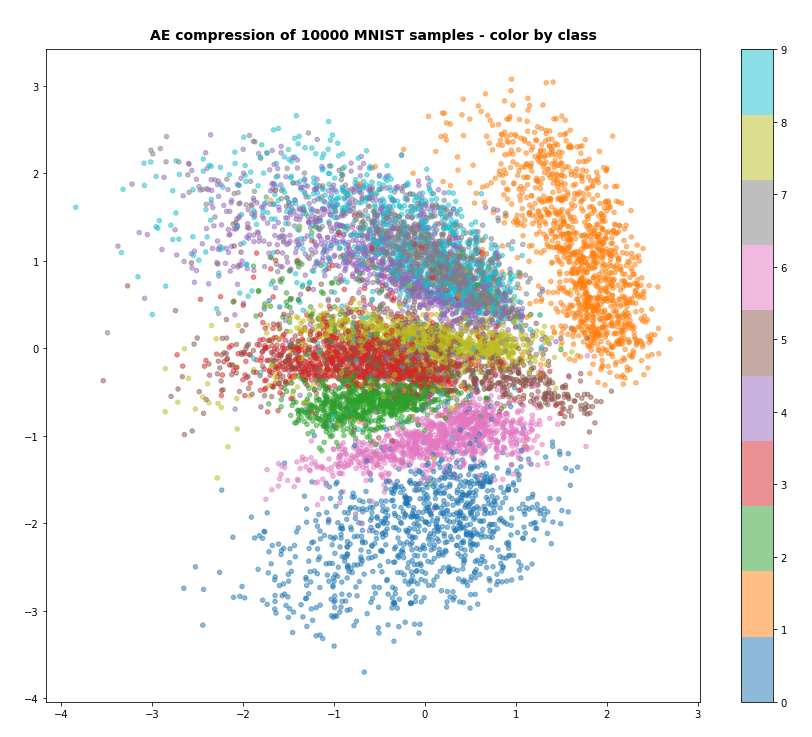# Conclusion

In this post we have used a standard option of Keras to define (eager execution compatible) loss functions. We transferred the KL loss related tensors „mu“ and „logvar“ to the Decoder and used them as different output tensors of our VAE-model. We needed to provide some dummy „y_true“ tensors to the cost functions. The approach is a bit complicated, but it is working under eager execution conditions and it does not reduce performance.
It also provided us with some insights into coupled „multi-input/multi-output models“ and cost handling for each of the outputs.

Still, this interesting approach appears as an overkill for handling the KL loss. In the next post

Variational Autoencoder with Tensorflow 2.8 – VII – KL loss via model.add_loss()

I shall turn to a seemingly much lighter approach which will use the model.add_loss() functionality of Keras.

Ceterum censeo: The worst living fascist and war criminal today, who must be isolated, denazified and imprisoned, is the Putler.

# Problems with upgrading Cyrus based IMAP servers to Opensuse Leap 15.3

I use 2 Mail servers whose IMAP components are based on Cyrus. The mail servers are major parts of KVM/Qemu based VMs. The OS of these VMs over more than a decade was some edition of Opensuse Linux (after SLES got too expensive for a Freelancer). Authorization to access the IMAP-servers is granted by a separate LDAP server via an SASL interface. All clients access the mail servers via a TLS connection. The communication to the LDAP system uses TLS, too. TLS connections are handled by SSSD which in turn uses PAM.

The configuration of all involved clients and servers is a bit tricky – and all components have special settings to interact smoothly. So I was always happy when upgrade processes of the servers respected my settings and things went smoothly. This was not always the case, but at least the main components survived the upgrade processes. But NOT this time.

The Leap 15.3 repositories do not contain Cyrus packages any longer! And I became aware of this when it was too late. Also the SLES update repositories available after the upgrade did not contain any Cyrus packages. After the upgrade the IMAP components of my mail servers were annihilated. Not funny at all!

Fortunately, I had backuped my VMs – and could restore them to bridge the time when I had to solve the problem. Afterward I spent some hours to try to reconstruct a running Cyrus configuration on the upgraded Leap 15.3 version of the mail server VM.

I got a suitable version of a Cyrus package which works with Leap 15.3 versions from the following repository :
download.opensuse.org / repositories / server: / mail / 15.3/.

However, while the installation after some changes of the configuration file worked well locally, I could not get access to it from external clients. In Kmail I got the message that the server did not support any security mechanisms. But STARTTLS should have worked! I checked the SSSD configuration, checked the imapd.config, nsswitch, ldap.config and certificate references. All OK.

I found the solution after having read some of my own old blog posts. The Leap upgrade had brutally deleted my carefully crafted PAM files „imap“ and „smtp“ in „/etc/pam.d/“. This has happened before. See:

So: Keep backpus of your PAM configuration if you have some complicated TLS-interactions between your Opensuse machines!

And start acquiring knowledge on Dovecot and the migration from Cyrus to Dovecot. Who knows when Cyrus disappears from all SuSE Repositories. And be prepared for problems with Cyrus and Leap 15.4, too.

I find it also frustrating that „https://doc.opensuse.org/release-notes/x86_64/openSUSE/Leap/15.3/“ does not explicitly state that the package „cyrus_imapd“ was removed. The information refers to changes in „cyrus-sasl“ – but this is a different package. Which ironically still exists (though modified) …

But I am too old to explode just because of the lack of important information …